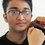# Need Help In Number Theory

If we divide all the numbers from $1$ to $1000$ by $7$.For how many numbers will the remainder be $3$ ?Note by Fazla Rabbi
6 years, 7 months ago

This discussion board is a place to discuss our Daily Challenges and the math and science related to those challenges. Explanations are more than just a solution — they should explain the steps and thinking strategies that you used to obtain the solution. Comments should further the discussion of math and science.

When posting on Brilliant:

• Use the emojis to react to an explanation, whether you're congratulating a job well done , or just really confused .
• Ask specific questions about the challenge or the steps in somebody's explanation. Well-posed questions can add a lot to the discussion, but posting "I don't understand!" doesn't help anyone.
• Try to contribute something new to the discussion, whether it is an extension, generalization or other idea related to the challenge.

MarkdownAppears as
*italics* or _italics_ italics
**bold** or __bold__ bold
- bulleted- list
• bulleted
• list
1. numbered2. list
1. numbered
2. list
Note: you must add a full line of space before and after lists for them to show up correctly
paragraph 1paragraph 2

paragraph 1

paragraph 2

[example link](https://brilliant.org)example link
> This is a quote
This is a quote
    # I indented these lines
# 4 spaces, and now they show
# up as a code block.

print "hello world"
# I indented these lines
# 4 spaces, and now they show
# up as a code block.

print "hello world"
MathAppears as
Remember to wrap math in $$ ... $$ or $ ... $ to ensure proper formatting.
2 \times 3 $2 \times 3$
2^{34} $2^{34}$
a_{i-1} $a_{i-1}$
\frac{2}{3} $\frac{2}{3}$
\sqrt{2} $\sqrt{2}$
\sum_{i=1}^3 $\sum_{i=1}^3$
\sin \theta $\sin \theta$
\boxed{123} $\boxed{123}$

Sort by:

hint:all such number will form an A.P wih comon diffrence 7.

- 6 years, 7 months ago

Note that every integer of the form $7k+3$ is a number that gives a remainder $3$ when divided $7$. $...(1)$

Equally importantly, whenever an integer divided by $7$ gives a remainder of $3$, it must be of the form $7k+3$. $...(2)$

Let $x$ be such an integer.

So, $1\leq x \leq 1000$ $\Leftrightarrow 1 \leq 7k+3 \leq 1000$ [ For some integer k] $\Leftrightarrow -2 \leq 7k \leq 997$ $\Leftrightarrow \frac {-2}{7} \leq k \leq \frac {1000}{7}$

There are $143$ integer solutions to this inequality from $0$ to $142$. From statement $(1)$, we can say, for each $k$ we can have a corresponding x satisfying the condition in the problem and from statement $(2)$, we can say, there is no other $x$'s other than these which will satisfy the condition. So there are $143$ integers from $1$ to $1000$ which give a remainder of $3$ when divided by $7$.

Hope this somewhat helps.

- 6 years, 7 months ago

You could condense your first two statements by saying an integer divided by 7 gives a remainder of 3 if and only if the number is of the form $7k+3$.

- 6 years, 7 months ago

Right, but the meaning of "if and only if" sometimes isn't clear to many people. They confuse it with only "if". That's why I did it.

- 6 years, 7 months ago

Try it for small numbers (say 1-30) and look for a pattern.

- 6 years, 7 months ago

Different hint: Consider $1\rightarrow 1000\pmod{7}$. How many numbers $1\le x\le 1000$ are there such that $x\equiv \pmod{7}$?

- 6 years, 7 months ago

Hint: All such numbers will be of form $7k+3$ with $0 \leq k \leq \lfloor \frac{1000}{7}\rfloor$ (As $142 \times 7 + 3 < 1000$)

- 6 years, 7 months ago

143

- 6 years, 7 months ago

We know that no. should be of the form (7x+3). First no.should be 3 and like this last no. should be 994+3 = 997 . That makes an AP with a =3, last term L =997 and common difference d =7. So, L =a + (n-1)d , where n is the no. of terms
997 =3 + (n-1)* 7, 994= (n-1)* 7, (n-1)= 142, n = 143.

- 6 years, 7 months ago

good job......solved problem in classical and simplest way unlike dat pappe

- 6 years, 7 months ago

thanks

- 6 years, 7 months ago

Explain how. This is not a question. Someone has posted this since he/she needs help in this.

- 6 years, 7 months ago